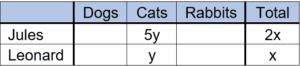# PCAT Quantitative Reasoning – Pet Ownership Word Problem

Jules and Leonard each owns two types of animals. Jules has twice as many pets and 5 times as many cats as Leonard does. Leonard also owns 2 dogs. The number of rabbits that Jules owns is equal to the number of cats owned by Leonard. How many total pets do Jules and Leonard have combined?

1. 3
2. 6
3. 9
4. 12
##### Click for Explanation

C is correct. One way to solve this problem is to create a table. The only pets mentioned are dogs, cats, and rabbits:We are told that Jules has twice as many pets as Leonard does. Let’s define the total number of pets owned by Leonard as x, and then we can define the number of pets owned by Jules as 2x. We are also told that Jules owns 5 times as many cats as Leonard does, so we can define these terms as 5y and y, respectively:Then we are told that Leonard owns two dogs. Since each person only owns two types of animals, we can conclude that Leonard owns 0 rabbits.We are then told that Jules owns as many rabbits as Leonard owns cats. Since Leonard owns y cats, then Jules must own y rabbits. Jules can only own two types of animals, so he must own 0 dogs.Now we can set up two equalities and solve for x and y!

Jules: 0 + 5y + y = 2x Leonard: 2 + y + 0 = x

6y = 2x

x = 3y

Plugging this into the equation for Leonard’s pets:

2 + y + 0 = 3y

2 = 2y

y = 1

We’re close, but we’re not done yet! Remember that we were asked to find the total number of pets owned by Jules and Leonard combined. First, we will solve for x and then plug that into the equation for the total number of pets:

x = 3y

y = 1

x = 3(1) = 3

Total = 2x + x = 3x = (3)(3) = 9

#### Submit a Comment

This site uses Akismet to reduce spam. Learn how your comment data is processed.